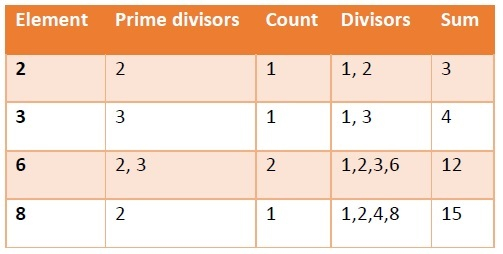# Program to find maximum size of any sequence of given array where every pair is nice in Python

Suppose we have a sequence nums of size n. We have to find the maximum size of subsequence of nums in which every pair (p, q) is a nice pair? A pait is said to be nice pair if and only if it holds at least one of these conditions: 1. The parity of the number of distinct prime divisors of p is equal to that of b. For example, the value 18 has two distinct prime divisors: 2 and 3. 2. The parity of the sum of all positive divisors of p is same as q.

So, if the input is like nums = [2,3,6,8], then the output will be 3To solve this, we will follow these steps −

• n := size of nums
• Define three empty lists cnt, total, result
• for each i in nums, do
• count := 0, tot := 0
• prime := a new list
• for each j in nums, do
• if (j mod k for all k in range 2 to j) is true, then
• insert j at the end of prime
• for each j in prime, do
• if i mod j is 0, then
• count := count + 1
• if count is even, then
• insert 'odd' at the end of cnt
• otherwise,
• insert 'even' at the end of cnt
• for j in range 1 to i, do
• if i mod j is same as 0, then
• tot := tot + j
• if tot is odd, then
• insert 'odd' at the end of total
• otherwise,
• insert 'even' at the end of total
• for i in range 0 to n-2, do
• for j in range i+1 to n - 1, do
• if cnt[i] is same as cnt[j] or total[i] is same as total[j], then
• insert nums[i] at the end of result
• if j is same as n-2, then
• insert nums[j] at the end of result
• result := a new list from a new set from result
• return size of result

## Example

Let us see the following implementation to get better understanding −

def solve(nums):
n = len(nums)
cnt = []
total = []
result = []
for i in nums:
count = 0
tot = 0

prime = []
for j in nums:
if all(j % k for k in range(2, j)) == True:
prime.append(j)

for j in prime:
if i % j == 0:
count += 1
if count % 2:
cnt.append('odd')
else:
cnt.append('even')

for j in range(1,i+1):
if i % j == 0:
tot += j

if tot % 2:
total.append('odd')
else:
total.append('even')

for i in range(n-1):
for j in range(i+1, n):

if cnt[i] == cnt[j] or total[i] == total[j]:
result.append(nums[i])

if j == n-1:
result.append(nums[j])

result = list(set(result))
return len(result)

nums = [2,3,6,8]
print(solve(nums))

## Input

15, 3, 8


## Output

3